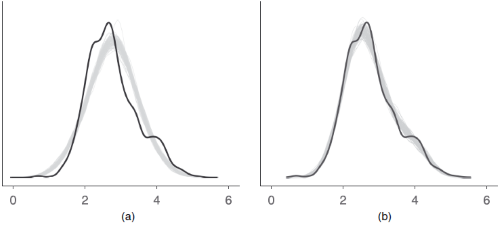Posterior predictive checks mean "simulating replicated data under the fitted model and then comparing these to the observed data" (Gelman and Hill, 2007, p. 158). Posterior predictive checks can be used to "look for systematic discrepancies between real and simulated data" (Gelman et al. 2014, p. 169).

performance provides posterior predictive check methods for a variety of frequentist models (e.g., lm, merMod, glmmTMB, ...). For Bayesian models, the model is passed to bayesplot::pp_check().

## Usage

check_predictions(object, ...)

# S3 method for default
check_predictions(
object,
iterations = 50,
check_range = FALSE,
re_formula = NULL,
bandwidth = "nrd",
type = "density",
verbose = TRUE,
...
)

posterior_predictive_check(object, ...)

check_posterior_predictions(object, ...)

## Arguments

object

A statistical model.

...

Passed down to simulate().

iterations

The number of draws to simulate/bootstrap.

check_range

Logical, if TRUE, includes a plot with the minimum value of the original response against the minimum values of the replicated responses, and the same for the maximum value. This plot helps judging whether the variation in the original data is captured by the model or not (Gelman et al. 2020, pp.163). The minimum and maximum values of y should be inside the range of the related minimum and maximum values of yrep.

re_formula

Formula containing group-level effects (random effects) to be considered in the simulated data. If NULL (default), condition on all random effects. If NA or ~0, condition on no random effects. See simulate() in lme4.

bandwidth

A character string indicating the smoothing bandwidth to be used. Unlike stats::density(), which used "nrd0" as default, the default used here is "nrd" (which seems to give more plausible results for non-Gaussian models). When problems with plotting occur, try to change to a different value.

type

Plot type for the posterior predictive checks plot. Can be "density", "discrete_dots", "discrete_interval" or "discrete_both" (the discrete_* options are appropriate for models with discrete - binary, integer or ordinal etc. - outcomes).

verbose

Toggle warnings.

## Value

A data frame of simulated responses and the original response vector.

## Details

An example how posterior predictive checks can also be used for model comparison is Figure 6 from Gabry et al. 2019, Figure 6.The model shown in the right panel (b) can simulate new data that are more similar to the observed outcome than the model in the left panel (a). Thus, model (b) is likely to be preferred over model (a).

## Note

Every model object that has a simulate()-method should work with check_predictions(). On R 3.6.0 and higher, if bayesplot (or a package that imports bayesplot such as rstanarm or brms) is loaded, pp_check() is also available as an alias for check_predictions().

• Gabry, J., Simpson, D., Vehtari, A., Betancourt, M., and Gelman, A. (2019). Visualization in Bayesian workflow. Journal of the Royal Statistical Society: Series A (Statistics in Society), 182(2), 389–402. https://doi.org/10.1111/rssa.12378

• Gelman, A., and Hill, J. (2007). Data analysis using regression and multilevel/hierarchical models. Cambridge; New York: Cambridge University Press.

• Gelman, A., Carlin, J. B., Stern, H. S., Dunson, D. B., Vehtari, A., and Rubin, D. B. (2014). Bayesian data analysis. (Third edition). CRC Press.

• Gelman, A., Hill, J., and Vehtari, A. (2020). Regression and Other Stories. Cambridge University Press.